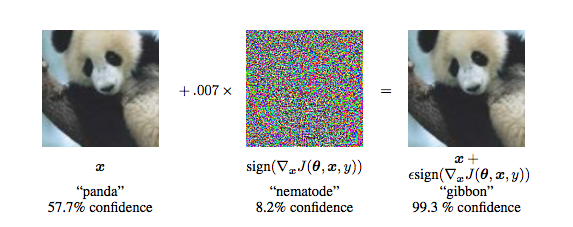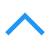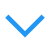# 生成式模型之 GAN

GAN的优化是一个极小极大博弈问题，公式如下：
$\underset{G}{\min} \: \underset{D}{\max}V(D,G) =\mathbb E_{x\sim p_{data}(x)}[logD(x)]+\mathbb E_{z\sim p_{z}(z)}[log(1-D(G(z)))]$

\begin{align} V(G,D)&=\int_x p_{data}(x)\log(D(x))dx+\int_zp_z(z)\log(1-D(g(z)))dz \\ &=\int_x [p_{data}(x)\log(D(x))+p_g(x)\log(1-D(x))]dx \end{align}

$D^*_G(x)={p_{data}(x)\over p_{data}(x)+p_g(x)}$

\begin{align} \underset{G}\min V(G,D^*_G) &= \int_x [p_{data}(x)\log{p_{data}(x)\over p_{data}(x)+p_g(x)}+p_g(x)\log{p_g(x)\over p_{data}(x)+p_g(x)}]dx \\ &= \mathbb E_{x\sim p_{data}}[\log{p_{data}(x)\over p_{data}(x)+p_g(x)}]+\mathbb E_{x\sim p_g}[\log{p_g(x)\over p_{data}(x)+p_g(x)}] \\ &= -\log 4+KL(p_{data}\|{p_{data}+p_g\over 2})+KL(p_g\|{p_{data}+p_g\over 2}) \\ &= -\log 4+2JS(p_{data}\|p_g) \end{align}

JS散度：$JS(P\|Q)={1\over 2}KL(P\|{P+Q\over 2})+{1\over 2}KL(Q\|{P+Q\over 2})$

JS散度具有对称性，而KL没有。

## f-GAN

### f-divergence

P和Q是两个分布,p(x),q(x)是x的分布概率
$D_f(P||Q)=\int_x q(x)f({p(x)\over q(x)})dx$

$\forall x,p(x)=q(x)$时,$D_f(P||Q)$具有最小值0.

$f(x)=x\log x$时,$D_f(P||Q)=\int_xp(x)\log({p(x)\over q(x)})dx$,即KL divergence.

$f(x)=-\log x$时,$D_f(P||Q)=\int_xq(x)\log({q(x)\over p(x)})dx$,即reverse KL divergence.

$f(x)=(x-1)^2$时,$D_f(P||Q)=\int_x{(p(x)-q(x))^2\over q(x)}dx$为Chi Square divergence.

### Fenchel Conjugate

$f^* (t)=\max_{x\in dom(f)}\{xt-f(x)\}$,且(f ) = f.

$f(x)=\max_{t\in dom(f^*)}\{xt-f^*(t)\}$,带入$D_f(P||Q)$得:
\begin{align} D_f(P||Q) &=\int_x q(x)f({p(x)\over q(x)})dx \\ &=\int_xq(x)(\max_{t\in dom(f^*)}\{{p(x)\over q(x)}t-f^*(t)\})dx \\ &=\max_D\int_x p(x)D(x)dx-\int_x q(x)f^*(D(x))dx \\ &\text{(t=D(x))} \end{align}

$D_f(P_{data}\|P_G)=\max_D\{E_{x\sim P_{data}}[D(x)]-E_{x\sim P_G}[f^*(D(x))]\}$

## WGAN

• Martin Arjovsky, Soumith Chintala, Léon Bottou, Wasserstein GAN, arXiv preprint, 2017
• Ishaan Gulrajani, Faruk Ahmed, Martin Arjovsky, Vincent Dumoulin, Aaron Courville,“Improved Training of Wasserstein GANs”, arXiv preprint, 2017